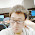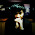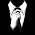## Combination Sum

Given a set of candidate numbers (C) and a target number (T), find all unique combinations in C where the candidate numbers sums to T.
The same repeated number may be chosen from C unlimited number of times.
Note:
• All numbers (including target) will be positive integers.
• Elements in a combination (a1a2, … , ak) must be in non-descending order. (ie, a1 ≤ a2 ≤ … ≤ ak).
• The solution set must not contain duplicate combinations.
For example, given candidate set 2,3,6,7 and target 7,
A solution set is:

[2, 2, 3]

### Analysis:

Because the problem is to get all the possible results, not the best or the number of result, thus we don't need to consider DP(dynamic programming), DFS is enough to handle it.

The idea is to scan from the first to the last element from the ordered array. (so first you need to sort the array) Then check every possible combination of numbers, note that each number can be used multiple times. So the looping in the function is not just from 0 to end, but for each element, keep use it until the sum is larger than the target.

All the possible selections of DFS are:
Each number in the array(multiple times can be used)

The termination condition of DFS is
1. the target ==0 , print list, return
2. the target < 0 return
3. start position >= array size return

Note: for python code, there is no "reference" (& in C++), when using the argument list, since "list" in python is mutable type, it can be considered as a reference in C++. But when you save the single result ("ress" in the code below), you have to use "ress[:]" to get the copy of each element in the variable, otherwise, when backtracking "ress", pop the last element in ress also pop the corresponding element in final result array "res".

### Code(C++):

class Solution {
public:
void dfs(vector<int> &candidates, int target, vector<vector<int> > &res, vector<int> &r, int i){
if (target<0){
return;
}else{
if (target==0){
res.push_back(r);
}else{
while (i<candidates.size() && target-candidates[i]>=0){
r.push_back(candidates[i]);
dfs(candidates,target-candidates[i],res,r,i);
i++;
r.pop_back();
}
}
}

}
vector<vector<int> > combinationSum(vector<int> &candidates, int target) {
// Start typing your C/C++ solution below
// DO NOT write int main() function
vector<vector<int> > res;
if (candidates.size()==0){return res;}
sort(candidates.begin(),candidates.end());
vector<int> r;
dfs(candidates,target,res,r,0);
return res;
}
};


### Code(Python):

class Solution:
def search(self, candidates, target, i, res, ress):
if target < 0:
return
else:
if target == 0:
res.append(ress[:]) #It is important to use "ress[:]" instead of "ress"
else:
while i < len(candidates) and target-candidates[i] >= 0:
ress.append(candidates[i])
self.search(candidates, target-candidates[i], i, res, ress)
i += 1
ress.pop(-1) #if use "ress", this will pop the elemtnes in res also

# @param {integer[]} candidates
# @param {integer} target
# @return {integer[][]}
def combinationSum(self, candidates, target):
res =[]
ress = []
self.search(sorted(candidates), target, 0, res, ress)
return res



1.请问 你这里的 r.pop_back(); 的作用是什么？

1.我明白了 2了。。谢谢

2.Is it possible to have duplicate results for the new version? Let's say my input is ([1, 1], 1)

1.Yes, I think so. I have run the test case ([1,1,],1) you provided, the output are two: {1}, and {1}.

2.Use a set instead of a list for the final result container would avoid the duplicated answers.

3.求助，请教大神，这道题目的time complexity怎么计算？我看网上有些答案是O(n!)，不知道如何算出来的。。。。谢谢啦！

4.there is a error in it, u have to remove the duplicate elements in it